Question

# Conduct a test at the alpha equals0.01 level of significance by determining ​(a) the null and...

Conduct a test at the

alpha

equals0.01

level of significance by determining ​(a) the null and alternative​ hypotheses, ​(b) the test​ statistic, and​ (c) the​ P-value. Assume the samples were obtained independently from a large population using simple random sampling.

Test whether

p 1 greater than p 2

.

The sample data are

x 1 equals 126

​,

n 1 equals 248

​,

x 2 equals 138

​,

and

n 2 equals 316

.

Below are the null and alternative Hypothesis,
Null Hypothesis, H0: p1 = p2
Alternate Hypothesis, Ha: p1 > p2

b)

p1cap = X1/N1 = 126/248 = 0.5081
p1cap = X2/N2 = 138/316 = 0.4367
pcap = (X1 + X2)/(N1 + N2) = (126+138)/(248+316) = 0.4681

Test statistic
z = (p1cap - p2cap)/sqrt(pcap * (1-pcap) * (1/N1 + 1/N2))
z = (0.5081-0.4367)/sqrt(0.4681*(1-0.4681)*(1/248 + 1/316))
z = 1.69

c)

P-value Approach
P-value = 0.0455
As P-value >= 0.01, fail to reject null hypothesis.

#### Earn Coins

Coins can be redeemed for fabulous gifts.

Similar Homework Help Questions
• ### Conduct a test at the a=0.01 level of significance by determining (a) the null and alternative...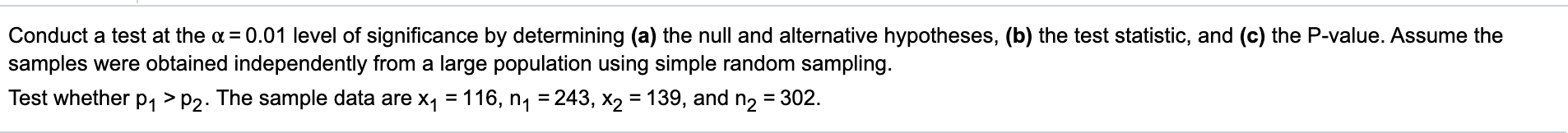Conduct a test at the a=0.01 level of significance by determining (a) the null and alternative hypotheses, (b) the test statistic, and (c) the P-value. Assume the samples were obtained independently from a large population using simple random sampling. Test whether P1 >P2. The sample data are x1 = 116, n1 = 243, X2 = 139, and n2 = 302.

• ### Conduct a test at the a=0.05 level of significance by determining (a) the null and alternative...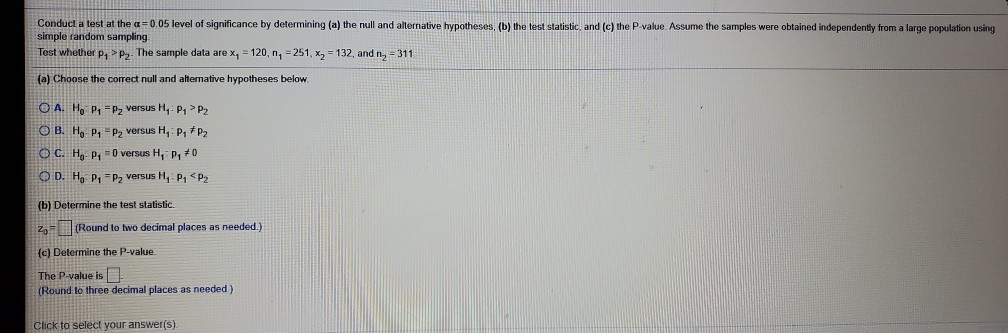Conduct a test at the a=0.05 level of significance by determining (a) the null and alternative hypotheses, (b) the test statistic, and (c) the P-value. Assume the samples were obtained independently from a large population using simple random sampling. Test whether pa > P2. The sample data are x = 120, n, 251, x2 = 132 and n, -311 (a) Choose the correct null and alternative hypotheses below O A. Ho P1 =Pz versus H, PP2 OB. Ho P, =...

• ### 5. Conduct a test at the a=0,01 level of significance by determining (a) the null and...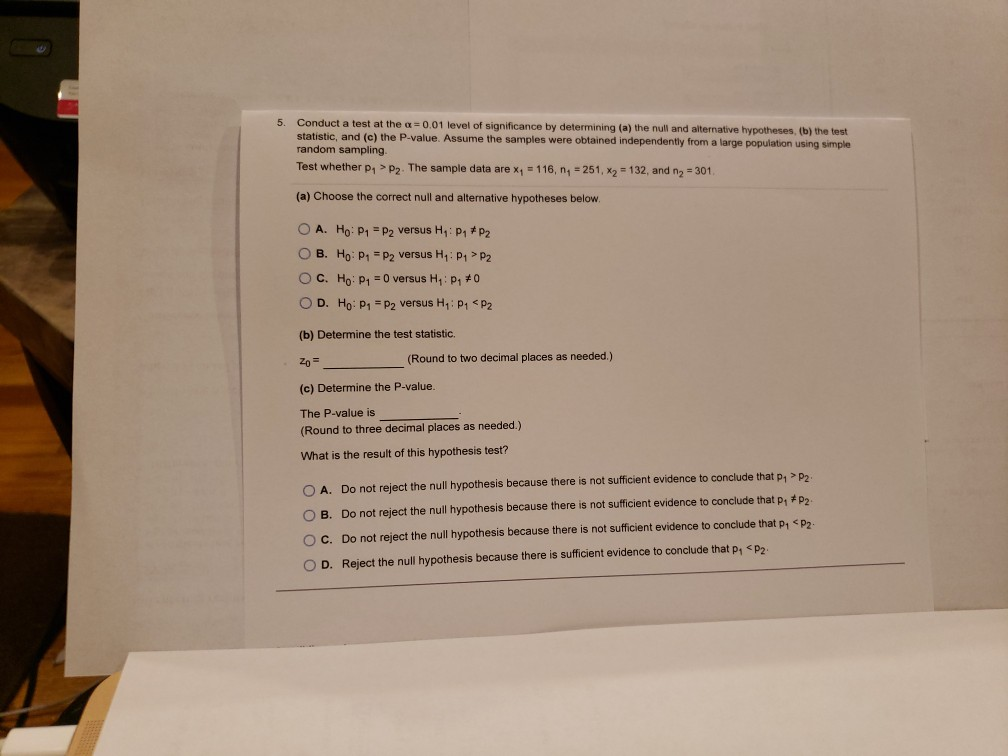5. Conduct a test at the a=0,01 level of significance by determining (a) the null and alternative hypotheses, (b) the test statistic, and (c) the P-value. Assume the samples were obtained independently from a large population using simple random sampling. Test whether P, > P2. The sample data are xy = 116, n = 251, x2 = 132, and n2 = 301 (a) Choose the correct null and alternative hypotheses below. O A. Ho: P1 = P2 versus H: PHP2...

• ### Conduct the following test at the a = 0.10 level of significance by determining (a) the...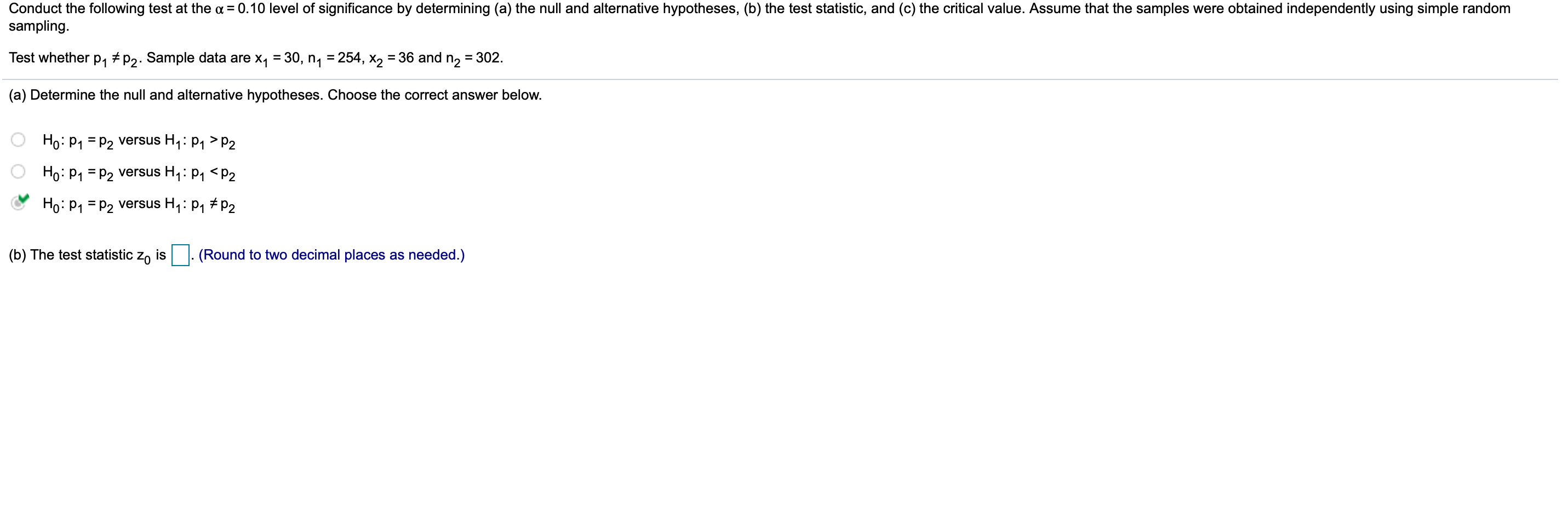Conduct the following test at the a = 0.10 level of significance by determining (a) the null and alternative hypotheses, (b) the test statistic, and (c) the critical value. Assume that the samples were obtained independently using simple random sampling. Test whether P1 7P2. Sample data are X7 = 30, ny = 254, X2 = 36 and n2 = 302. (a) Determine the null and alternative hypotheses. Choose the correct answer below. Ho: P1 = P2 versus Hy: P1 >P2...

• ### Conduct the following test at the alphaequals0.05 level of significance by determining ​(a) the n...

Conduct the following test at the alphaequals0.05 level of significance by determining ​(a) the null and alternative​ hypotheses, ​(b) the test​ statistic, and​ (c) the​ P-value. Assume that the samples were obtained independently using simple random sampling. Test whether p 1 not equals p 2. Sample data are x 1 equals 30​, n 1 equals 254​, x 2 equals 38​, and n 2 equals 301. ​(a) Determine the null and alternative hypotheses. Choose the correct answer below. A. Upper H...

• ### Conduct a test at the a = 0.10 level of significance by determining (a) the null...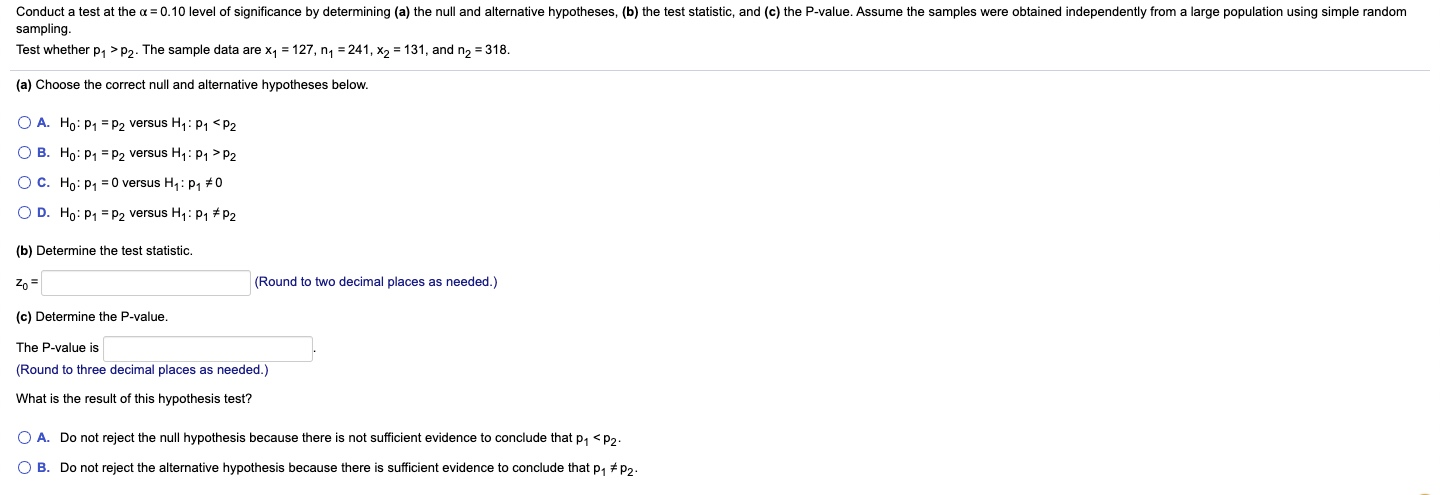Conduct a test at the a = 0.10 level of significance by determining (a) the null and alternative hypotheses, (b) the test statistic, and (c) the P-value. Assume the samples were obtained independently from a large population using simple random sampling. Test whether 21 > P2. The sample data are x1 = 127, ny = 241, X2 = 131, and n2 = 318. (a) Choose the correct null and alternative hypotheses below. O A. Ho: P1 = P2 versus Hy:...

• ### Conduct the following test at the a = 0.05 level of significance by determining (a) the...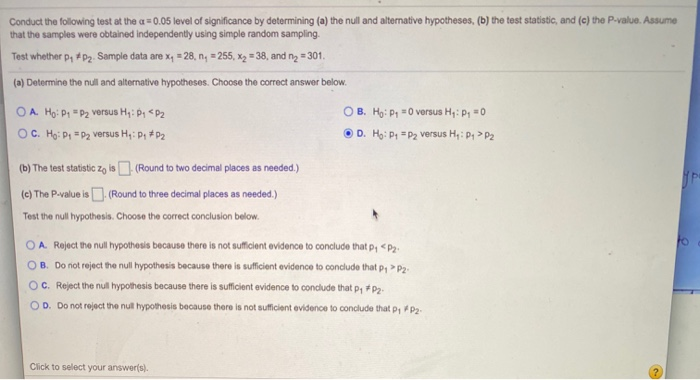Conduct the following test at the a = 0.05 level of significance by determining (a) the null and alternative hypotheses, (b) the test statistic, and (c) the P-value. Assume that the samples were obtained independently using simple random sampling. Test whether pe p2. Sample data are xy = 28, n, = 255, x2 = 38, and nz = 301 (a) Determine the null and alternative hypotheses. Choose the correct answer below. O A. Ho: P." P2 versus H: PP2 OC....

• ### Conduct the following test at the a = 0.05 level of significance by determining (a) the...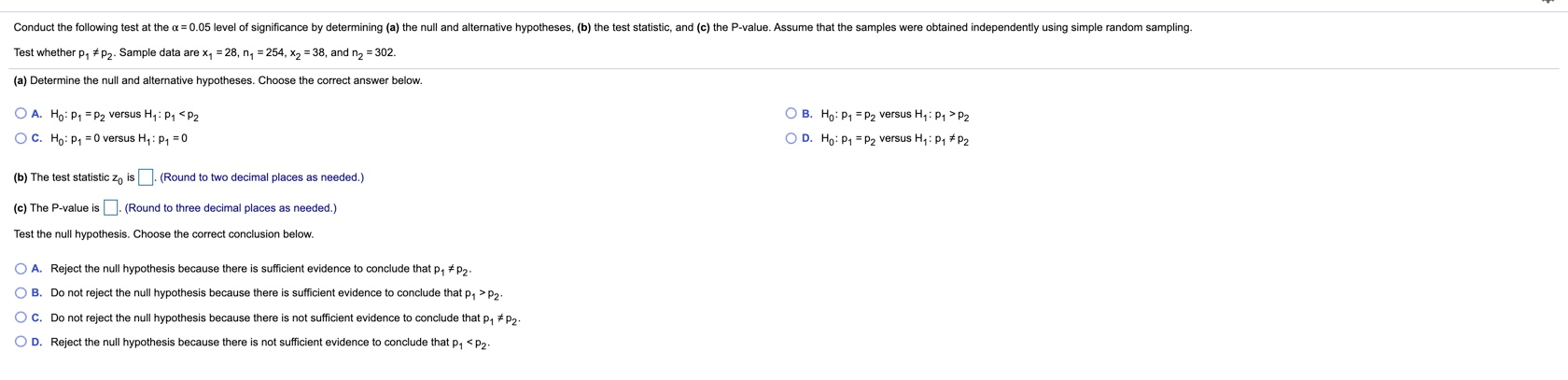Conduct the following test at the a = 0.05 level of significance by determining (a) the null and alternative hypotheses, (b) the test statistic, and (c) the P-value. Assume that the samples were obtained independently using simple random sampling. Test whether P1 * P2. Sample data are x, = 28, ny = 254, X2 = 38, and n2 = 302. (a) Determine the null and alternative hypotheses. Choose the correct answer below. OB. Ho: P = P2 versus HP1 P2...

• ### Conduct the following test at the a = 0.01 level of significance by determining (a) the...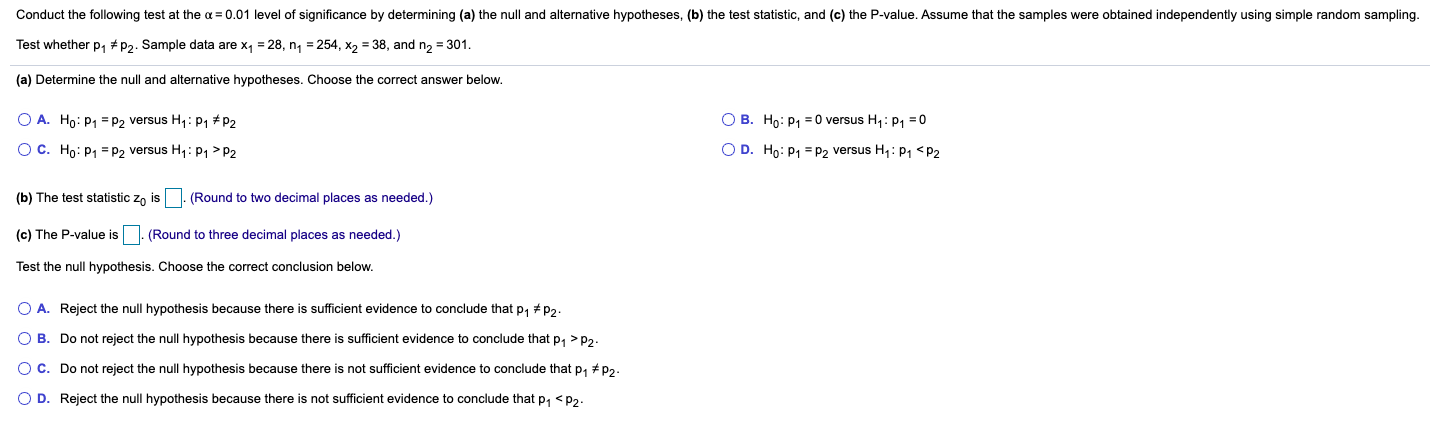Conduct the following test at the a = 0.01 level of significance by determining (a) the null and alternative hypotheses, (b) the test statistic, and (c) the P-value. Assume that the samples were obtained independently using simple random sampling. Test whether P1 * P2. Sample data are xy = 28, n = 254, X2 = 38, and n2 = 301. (a) Determine the null and alternative hypotheses. Choose the correct answer below. O A. Ho: P1 = P2 versus Hy:...

• ### Conduct the following test at the a = 0.01 level of significance by determining (a) the...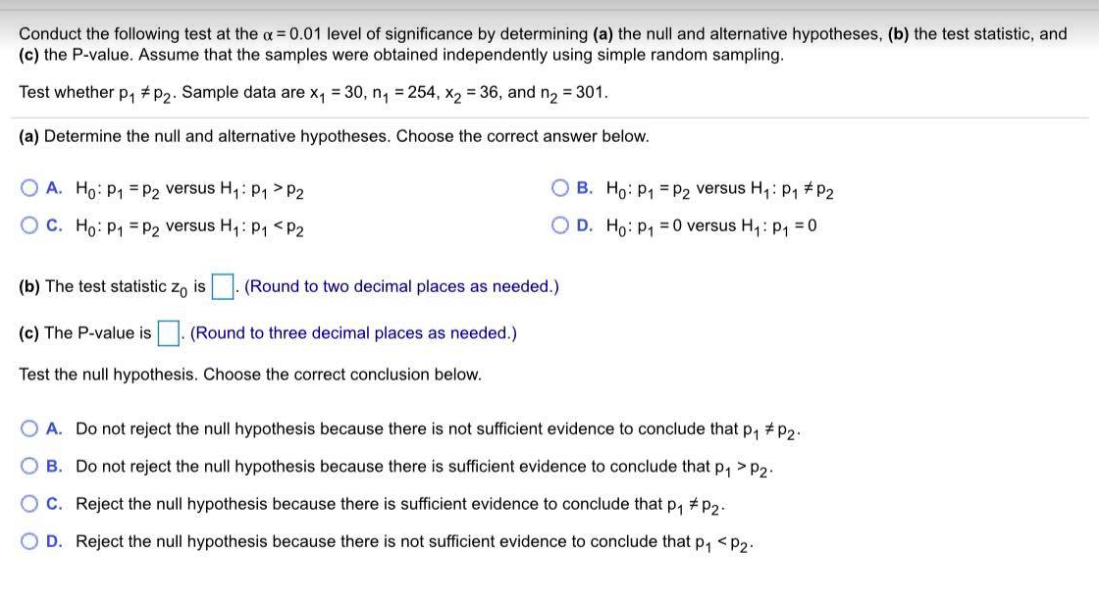Conduct the following test at the a = 0.01 level of significance by determining (a) the null and alternative hypotheses, (b) the test statistic, and (c) the P-value. Assume that the samples were obtained independently using simple random sampling. Test whether p1 #P2. Sample data are X7 = 30, n = 254, X2 = 36, and n2 = 301. (a) Determine the null and alternative hypotheses. Choose the correct answer below. O A. Ho: P1 = P2 versus H: P1>P2...